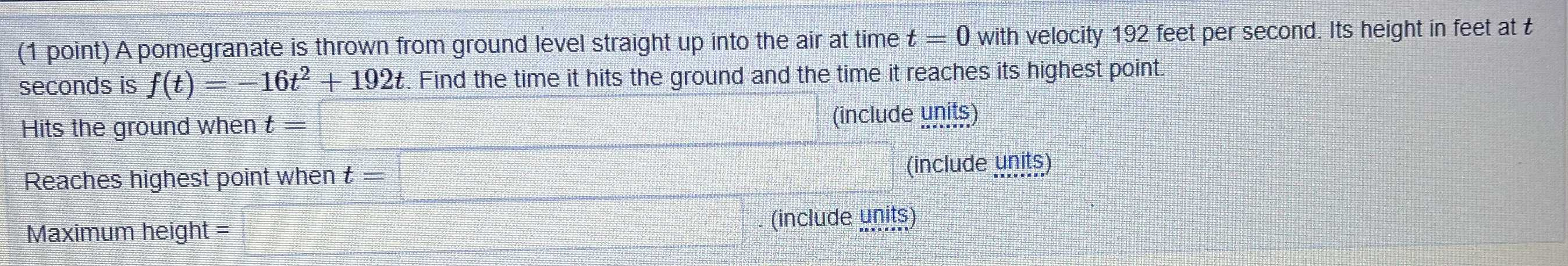### ¿Todavía tienes preguntas de matemáticas?

Pregunte a nuestros tutores expertos
Algebra
Pregunta(1 point) A pomegranate is thrown from ground level straight up into the air at time $$t = 0$$ with velocity $$192$$ feet per second. Its height in feet at $$t$$ seconds is $$f ( t ) = - 16 t ^ { 2 } + 192 t$$ . Find the time it hits the ground and the time it reaches its highest point.

Hits the ground when $$t =$$

Reaches highest point when $$t =$$

Maximum height $$=$$